# Composite Linear/Nonlinear Model

According to Equation (6.25), the time evolution of the reconnected magnetic flux (in a frame of reference that co-rotates with the magnetic island chain) in the linear regime is governed by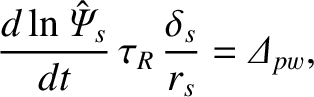(9.6)

where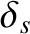is the linear layer width. The previous equation can be rearranged to give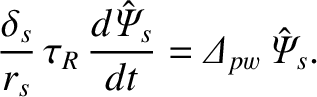(9.7)

Now, given that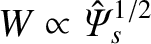[see Equation (8.1)], the Rutherford island width evolution equation, (9.5), can be rewritten in the form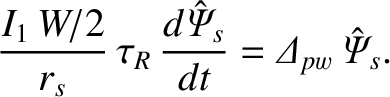(9.8)

It can be seen, via a comparison between the previous two equations, that a nonlinear magnetic island chain evolves in time in an analogous fashion to a linear layer whose width is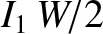. In other words, the essential nonlinearity in the nonlinear regime comes about because the effective layer width is amplitude dependent.

Now, Equation (9.7) is valid when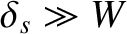(i.e., when the linear layer width is much greater than the island width), whereas Equation (9.8) is valid in the opposite limit. This observation allows us to formulate a composite time evolution equation that encompasses both the linear and the nonlinear regimes [3,4,8,10]: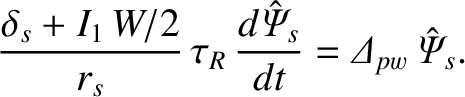(9.9)

The previous equation can also be written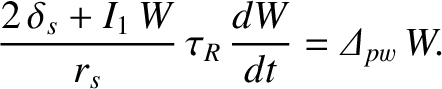(9.10)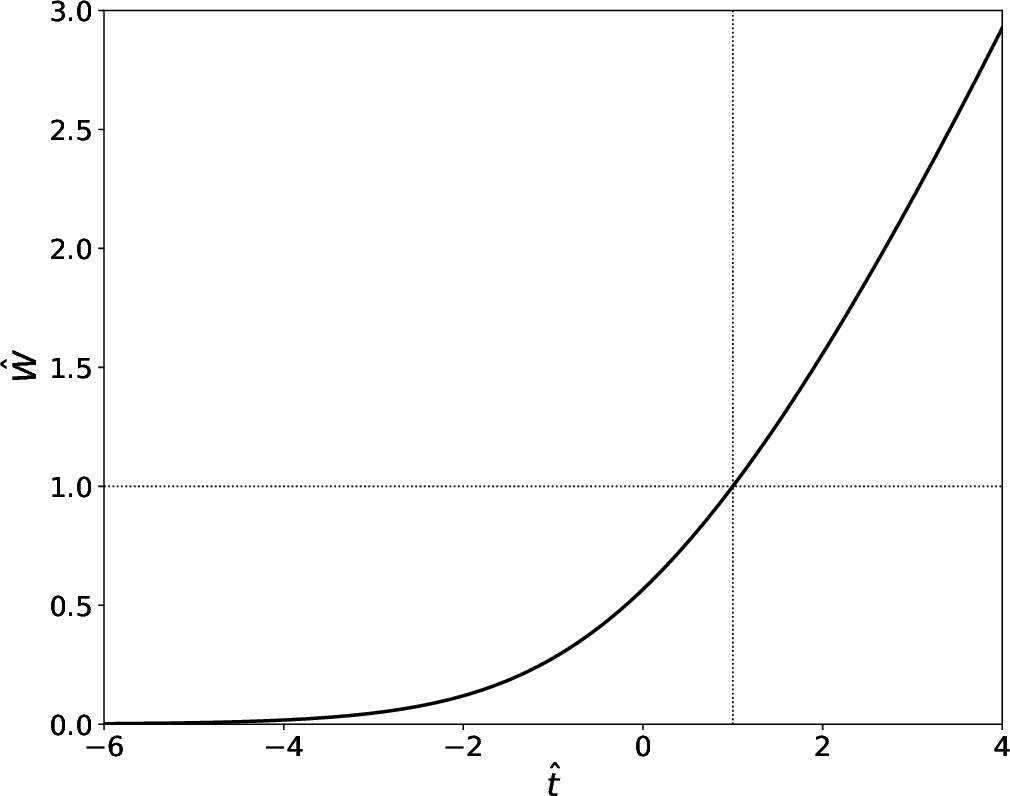Let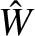(9.11)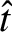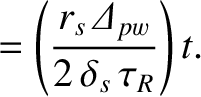(9.12)

Equation (9.10) transforms into(9.13)

which can be solved to give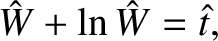(9.14)

assuming that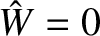at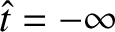. Figure 9.1 shows the time evolution of the magnetic island width predicted by the previous equation. It can be seen that the evolution makes a smooth transition from exponential growth when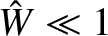(i.e., when the island width is much less than the linear layer width) to algebraic growth when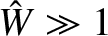(i.e., when the island width is much greater than the linear layer width).